• matlab电偶极子电视电场图仿真平面图以及三维图，形象直观展现出电偶极子在空间中电场以及电势分布，分方便的学习和理解
• 电偶极子周围电场电势分布的MATLAB三维模拟Matlab可以用于模拟四维场的效果，本文以电偶极子的电场和电势场为例展示MATLAB在该方面领域的应用。摘要电偶极子由一个正电荷和一个负电荷组成，其电场和空间电势分布与单...

电偶极子周围电场电势分布的MATLAB三维模拟
Matlab可以用于模拟四维场的效果，本文以电偶极子的电场和电势场为例展示MATLAB在该方面领域的应用。
摘要
电偶极子由一个正电荷和一个负电荷组成，其电场和空间电势分布与单电荷相比具有一些重要的特性。本文将给出电场和电势场的近似表达式。这两个量的精确表达式是复杂的，不太常用，因此 MATLAB 被采用来操作精确值和绘制三维分布的远区域， 近区域和一侧区域的电偶极子
关键词： 电偶极子 ， 电势， 能量密度， 电场， MATLAB
引言
由正电荷和负电荷等于q组成的系统，距离为d，称为电偶极子。对于电偶极子，我们定义了一个新的矢量，称为电偶极矩。电偶极子矩矢量 p 的大小是电荷 q 的大小，乘以它们之间的距离 d，P=qd。 向量方向从负电荷指向正电荷。电偶极子的电场和电势可以通过两个电荷的叠加来计算。这种方法很容易想出来，但有时很难计算结果。因此，我们可以使用合理的近似法来简化计算公式。电偶极子矩将用作新公式中的一个变量。
电偶极子的电势场
物理模型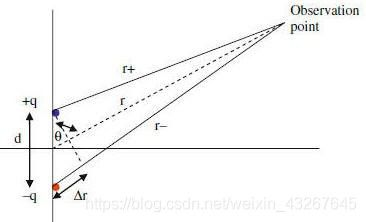我们可以利用两个电荷的电势叠加来获取太空中的电势。图1是一个电偶极子，其中心位于z轴上，两个电荷之间的距离为d，从点P到q和-q的距离为r+和**r-。
单个电荷的电势公式：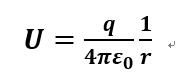则我们可以以此类推，空间中的电势的分布公式为：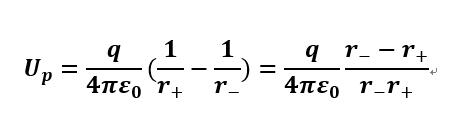当电偶极子中心到目标点的距离r远大于距离d时(实际上电偶极子默认的一个的定义就是这个)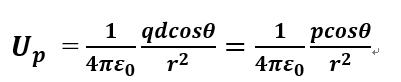其中 r- =Δ \DeltaΔr= r d c o s θ r d cos\thetardcosθ, 1/(r+r-) 可以认为等于 1 / r 2 1/r^{2}1/r2。因为 r >>Δ \DeltaΔr 起。从这个公式中我们可以知道，电偶极子电位没有球形对称性，并且会以1 / r 2 1/r^{2}1/r2的速度下降，比单个电子的库仑定律计算出的电位快得多，后者的电位会以1 / r 1/r1/r的速度下降。
MATLAB 模拟
我们设置 +q 在 (1，0，0) 和 -q 在 (-1，0，0)，选择 1000 点在空间中，并计算他们的电势，绘制该1000个点用不同的颜色， 当颜色的波长较长(偏红)， 电位是正， 颜色的波长较短(偏蓝)， 电位是负。
我们首先考虑空间中电势的 远区场，并将采样点的范围设置为 -4 到 4。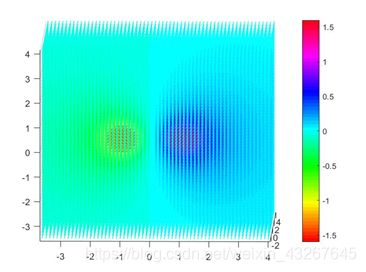在此图中，我们可以粗略看到电偶极子的电势分布与仅单个电子的电势分布不同。
但是，因为 MATLAB 必须为每个值分配颜色，因此当采样点接近电荷中心时，该值将远远大于远离电荷中心的值。
为了解决这个问题我们可以采用手动消除较大值的方法(比较原始的办法，若大家有更好的办法欢迎分享)。
具体操作为选择靠近电荷一定范围的电设置他们的电势为一个相对较小的值。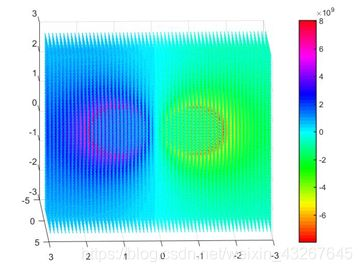我们最终得到如上效果图。
此时如果我们想更进一步看出电偶极子单侧电势分布与单个电子时的区别，我们就可以对单侧进一步模拟：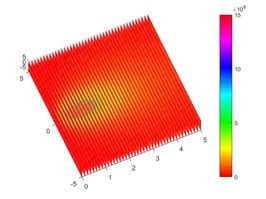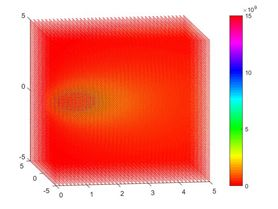效果非常明显。
MATLAB代码
x0=-4.5:0.15:4.5;
y0=-4.5:0.15:4.5;
z0=-4.5:0.15:4.5;%生成空间中226981个点
points1=zeros(61,61,61);
points2=zeros(3, 226981);%装入所有点的三个坐标的矩阵初始化
c=zeros(1, 226981);
q=1.6e-19;
pointA=[1 0 0]';
pointB=[-1 0 0]';%定义电荷位置
for i=1:61
for j=1:61
for k=1:61%这里用了点技术手段，为了把三维坐标放入二维的矩阵里
points2(:,k+61*(j-1)+ 3721*(i-1))=[x0(i),y0(j),z0(k)]';%点坐标矩阵
end
end
end
k=9e10;
%以上代码可以用linspace加meshgrid的组合代替，具体方法此处便不累述了
for p=1: 226981
c(p)=k*((1/(norm(points2(:,p)-pointA))-1/(norm(points2(:,p)-pointB))));%c矩阵用来储存每个点的电势值
if (norm(points2(:,p)-pointB)<1)%此处便是手动去掉过大或过小值的地方，1是调整半径
c(p)=-6e10;
end
% if (norm(points2(:,p)-pointA)<1)%此处便是手动去掉过大或过小值的地方，1是调整半径
% c(p)=6e10;%要画单侧的时候去掉这个if，双侧的时候保留这一块if语句
%end
% if (c(p)>0)%这是画一边的电势分布时用的
% c(p)=1e7;%就是把另一边儿的点值归为一个同号的值然后坐标设为原点
% points2(:,p)=[0 0 0]';%如果需要全局的，去掉该if便可
% end
end
scatter3(points2(1,:),points2(2,:),points2(3,:),2,c,'.')%电势值的大小用scatter3函数中的颜色参数来表示，2是点的大小参数
colorbar
电偶极子的电场
物理模型
我们可以利用这两个电荷的电场的叠加来获得空间中的电场，但是这种方法需要矢量操作，如果我们想要获得电场的分析表达式，这种方法可能很复杂。为了避免这种情况，我们选择计算电势场的梯度，以获得空间中的电偶极子电场分布。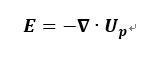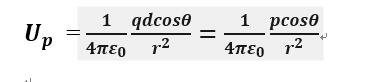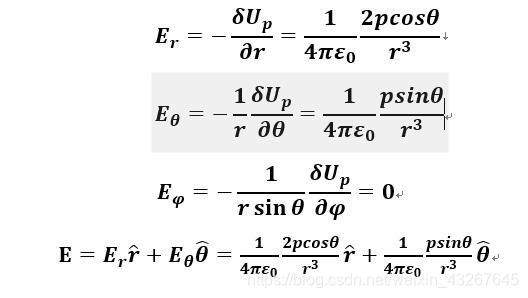这里附上一段如何推导球坐标系下的偏导公式的过程(转载自 从本质出发理解掌握三大坐标系下的三大方程(一)——梯度公式)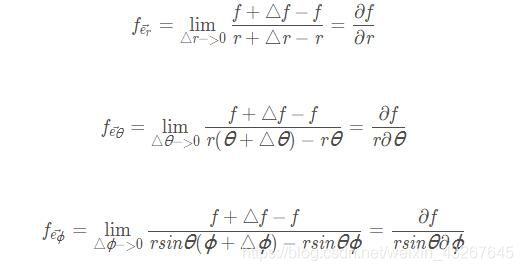MATLAB模拟
取1000点被收集于一个长度为6的正方体，这可以帮助我们得到远区场电场。电场图基于电势分布，因此与以前相似的电势图将用来显示更有效的结果。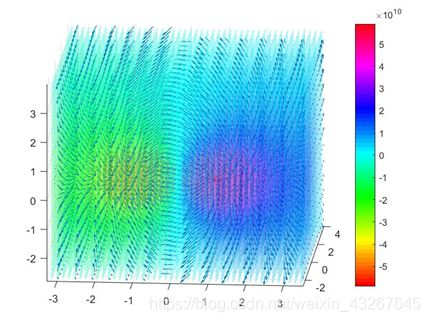我们可以用同样的方法求出近区场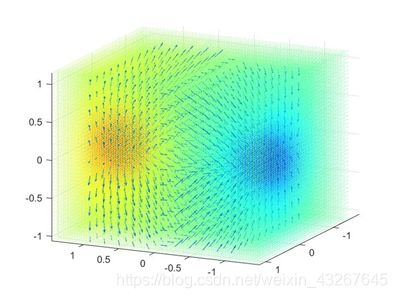单侧电场分布如下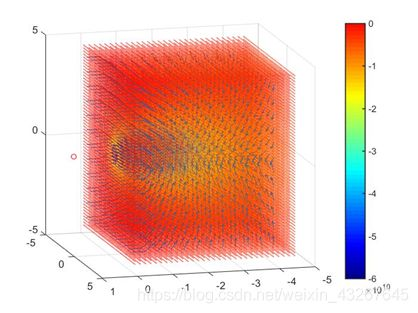MATLAB代码
%注意这个代码单独使用只能生成带箭头的图，与上一部分代码联合使用才能做出上面的图
x=linspace(-4, 0, 12);
y=linspace(-4, 4, 12);
z=linspace(-4, 4, 12);
[xx, yy, zz]=meshgrid(x, y,z);%此处便用了上面提到的linspace加meshgrid的组合
%xx,yy,zz均为10*10*10的三维数组，分别对应空间中1000个点的x y z坐标值
up=-9e10.*(1./sqrt((xx-1).^2+yy.^2+zz.^2)-1./sqrt((xx+1).^2+yy.^2+zz.^2));%up为每个点的电势值
hold on;
dx=0.5*dx./sqrt(dx.^2+dy.^2+dz.^2);%将梯度向量等长度化
dy=0.5*dy./sqrt(dx.^2+dy.^2+dz.^2);
dz=0.5*dz./sqrt(dx.^2+dy.^2+dz.^2);
quiver3(xx,yy,zz,dx, dy,dz);%该处用到quiver3画箭头专用函数
plot3(1,0,0,'o','color','r','MarkerSize',5)

展开全文• 以下是你的课程论文题目：用数值计算的方法研究三维空间中电偶极子的电场以及电势的分布。Matlab程序：k=1;q=1;d=0.01;h=0.8;[x,y]=meshgrid([-h:0.1:h]);%建立二维网格r2=sqrt((x-d/2).^2+y.^2+0.001);r1=sqrt((x+d...

以下是你的课程论文题目：
用数值计算的方法研究三维空间中电偶极子的电场以及电势的分布。
Matlab程序：
k=1;q=1;d=0.01;h=0.8;
[x,y]=meshgrid([-h:0.1:h]);%建立二维网格
r2=sqrt((x-d/2).^2+y.^2+0.001);r1=sqrt((x+d/2).^2+y.^2+0.001);
phi=k*q*(1./r2-1./r1);%电势计算公式
AE=sqrt(Ex.^2+Ey.^2);Ex=Ex./AE;Ey=Ey./AE;%场强归一化，使箭头等长CV=linspace(min(min(phi)),max(max(phi)),49);%产生49个电位值
subplot(1,2,1)
contour(x,y,phi,CV);%画电势图
hold on %在原图像上作图
plot([-h:0.01:h],0,'r-');%作出x轴
hold off%取消保持图像
axis([-h h -h h]);title('等势线');
subplot(1,2,2)
quiver(x,y,Ex,Ey,'k');%用箭头作出电场线图
hold on %在原图像上作图
plot([-h:0.01:h],0,'r-');%作出x轴
hold off%取消保持图像
axis([-h h -h h]);title('电场线');

展开全文• 通过编程计算电偶极子所在平面各点处电场强度一、电偶极子概念的引入 电偶极子(electric dipole)是两个相距很近的等量异号点电荷组成的系统。电偶极子的特征用电偶极距描述，其中是两点电荷之间的距离，和的方向规定...

通过编程计算电偶极子所在平面各点处电场强度
一、电偶极子概念的引入
电偶极子(electric dipole)是两个相距很近的等量异号点电荷组成的系统。电偶极子的特征用电偶极距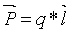描述，其中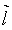是两点电荷之间的距离，和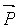的方向规定由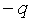指向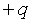。电偶极子在外电场中受力矩作用而旋转，使其电偶极矩转向外电场方向。电偶极矩就是电偶极子在单位外电场下可能受到的最大力矩，故简称电矩。如果外电场不均匀，除受力矩外，电偶极子还要受到平移作用。电偶极子产生的电场是构成它的正、负点电荷产生的电场之和。而本文主要研究由一对电偶极子在所在平面中各点产生的电场强度。
二、电偶极子所在平面特殊位置场强的计算及近似
(笔者注：本文推导所有静电力常量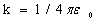，其中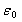为真空介电常数 )
1.电偶极子矢径延长线上的场强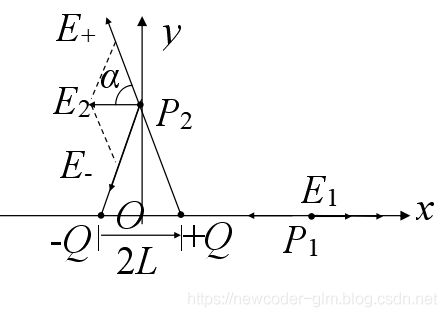如图所示，等量异号点电荷在轴线上的P1点产生的电场强度的方向相反，合场强沿x轴正向，大小为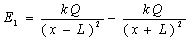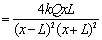取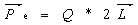，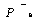称为电偶极矩，其方向从负电荷指向正电荷。
代入上式即可得到电偶极子矢径延长线上的场强关于电偶极矩的表达式
2.电偶极子中垂线上的场强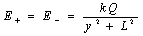，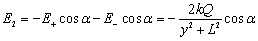当L << y时，合场强就是电偶极子场强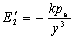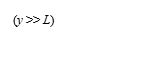当场点P2在y的负半轴上时，场强方向也沿x轴负方向，电偶极子场强可用矢量表示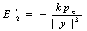3.任意位置场强计算
电偶极子的电场强度可用场强叠加原理求解，这里我通过电势梯度求解更简单。
如图所示，等量异号电荷在任意点P产生的电势为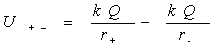其中，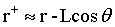，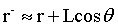由以上式子得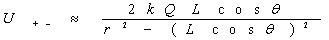利用x = r，y = r，将所得电势表达式分别对X 、Y求偏导乘-1得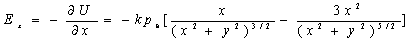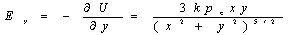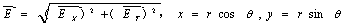，
得合场强为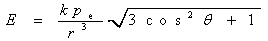电偶极子的合场强与距离的3次方成正比，还与方向有关，也容易得出：在距离一定时，连线上的场强最大，中垂线上的场强最小，最大值是最小值的2倍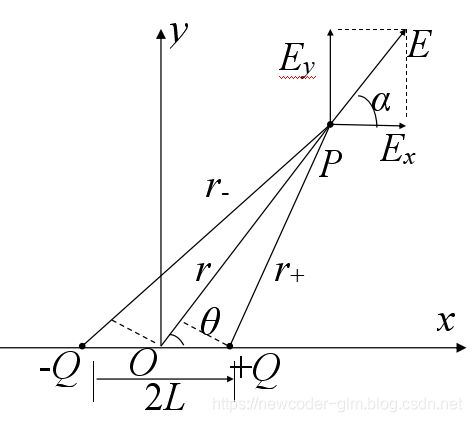三、利用MATLAB软件绘制电偶极子所在平面各点处电场线
Code：
(dojz.m)
clear;
clf;
q=2e-6;k=9e9;a=2.0;b=0;
x=-6:0.3:6;
y=x;
[X,Y]=meshgrid(x,y);
rp=sqrt((X-a).2+(Y-b).2);rm=sqrt((X+a).2+(Y+b).2);
%所求点到正负电荷的距离分别为rp和rm
V=qk(1./rp-1./rm);
%所求点电势
%根据该点电势对x、y求偏导的负值就是该点x、y方向场强
AE=sqrt(Ex.2+Ey.2);Ex=Ex./AE;Ey=Ey./AE;
cv=linspace(min(min(V)),max(max(V)),201);
contour(X,Y,V,cv,‘r-’)
%画图
title('电偶极子全平面场强分布图 '),hold on
quiver(X,Y,Ex,Ey,0.6,‘g’)
plot(a,b,‘bo’,a,b,‘gd’)
plot(-a,-b,‘bo’,-a,-b,‘w*’)
xlabel(‘X axis’);ylabel(‘Y axis’);
hold on
图像：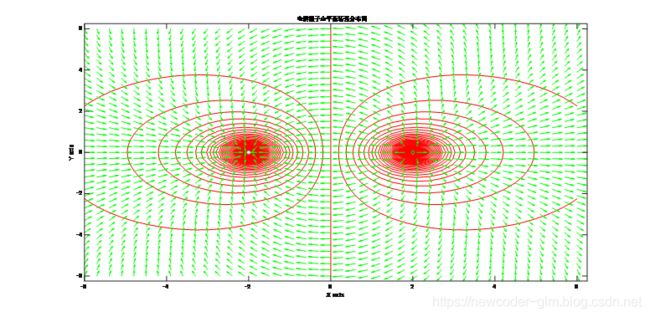四、课题总结
虽然通过微积分及初等数学、小量近似等方法理论上可以求解出任意静止电荷在平面内产生的电场，但对于电荷组成复杂的系统，我们还是比较倾向于采用计算机数学辅助软件求解，通过对MATLAB编写程序求解电偶极子所在平面各点处电场强度，既增强了编程能力，也对电偶极子这种经典的电荷模型有清晰的了解。

展开全文• matlab绘制三维电偶极子的电场和电势.zip
• Matlab具有丰富的计算功能和科学计算数据的可视化能力，特别是应用偏微分方程工具箱在大学物理电磁场的数值仿真中具有无比的优势。下文是在利用Matlab 软件仿真带电粒子在不同...运行结果为电偶极子的电场线与等势线
• ## 电偶极子的MATLAB场模拟

千次阅读 多人点赞 2019-12-11 15:10:11
电偶极子周围电场电势分布的MATLAB三维模拟 Matlab可以用于模拟四维场的效果，本文以电偶极子的电场和电势场为例展示MATLAB在该方面领域的应用。 摘要 电偶极子由一个正电荷和一个负电荷组成，其电场和空间电势分布...
电偶极子周围电场电势分布的MATLAB三维模拟
Matlab可以用于模拟四维场的效果，本文以电偶极子的电场和电势场为例展示MATLAB在该方面领域的应用。
摘要
电偶极子由一个正电荷和一个负电荷组成，其电场和空间电势分布与单电荷相比具有一些重要的特性。本文将给出电场和电势场的近似表达式。这两个量的精确表达式是复杂的，不太常用，因此 MATLAB 被采用来操作精确值和绘制三维分布的远区域， 近区域和一侧区域的电偶极子
关键词： 电偶极子 ， 电势， 能量密度， 电场， MATLAB
引言
由正电荷和负电荷等于q组成的系统，距离为d，称为电偶极子。对于电偶极子，我们定义了一个新的矢量，称为电偶极矩。电偶极子矩矢量 p 的大小是电荷 q 的大小，乘以它们之间的距离 d，P=qd。 向量方向从负电荷指向正电荷。电偶极子的电场和电势可以通过两个电荷的叠加来计算。这种方法很容易想出来，但有时很难计算结果。因此，我们可以使用合理的近似法来简化计算公式。电偶极子矩将用作新公式中的一个变量。
电偶极子的电势场
物理模型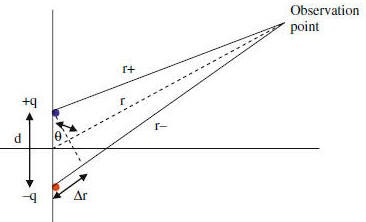我们可以利用两个电荷的电势叠加来获取太空中的电势。图1是一个电偶极子，其中心位于z轴上，两个电荷之间的距离为d，从点P到q和-q的距离为r+和**r-。 单个电荷的电势公式：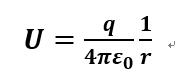则我们可以以此类推，空间中的电势的分布公式为：当电偶极子中心到目标点的距离r远大于距离d时（实际上电偶极子默认的一个的定义就是这个）其中 r- =

Δ

\Delta

r=

r

d

c

o

s

θ

r d cos\theta

, 1/（r+r-） 可以认为等于

1

/

r

2

1/r^{2}

。因为 r >>

Δ

\Delta

r 起。从这个公式中我们可以知道，电偶极子电位没有球形对称性，并且会以

1

/

r

2

1/r^{2}

的速度下降，比单个电子的库仑定律计算出的电位快得多，后者的电位会以

1

/

r

1/r

的速度下降。
MATLAB 模拟
我们设置 +q 在 （1，0，0） 和 -q 在 （-1，0，0），选择 1000 点在空间中，并计算他们的电势，绘制该1000个点用不同的颜色， 当颜色的波长较长（偏红）， 电位是正， 颜色的波长较短（偏蓝）， 电位是负。 我们首先考虑空间中电势的 远区场，并将采样点的范围设置为 -4 到 4。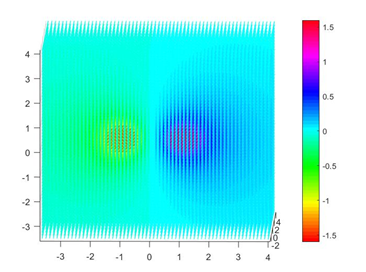在此图中，我们可以粗略看到电偶极子的电势分布与仅单个电子的电势分布不同。
但是，因为 MATLAB 必须为每个值分配颜色，因此当采样点接近电荷中心时，该值将远远大于远离电荷中心的值。
为了解决这个问题我们可以采用手动消除较大值的方法（比较原始的办法，若大家有更好的办法欢迎分享）。
具体操作为选择靠近电荷一定范围的电设置他们的电势为一个相对较小的值。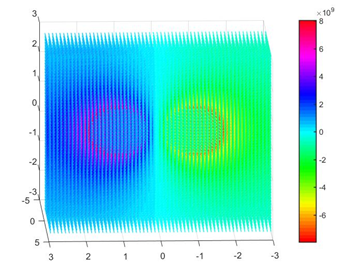我们最终得到如上效果图。
此时如果我们想更进一步看出电偶极子单侧电势分布与单个电子时的区别，我们就可以对单侧进一步模拟：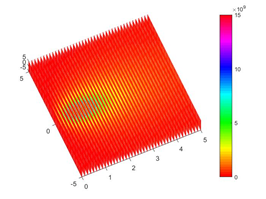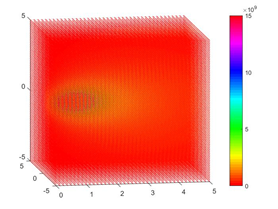效果非常明显。
MATLAB代码
x0=-4.5:0.15:4.5;
y0=-4.5:0.15:4.5;
z0=-4.5:0.15:4.5;%生成空间中226981个点
points1=zeros(61,61,61);
points2=zeros(3, 226981);%装入所有点的三个坐标的矩阵初始化
c=zeros(1, 226981);
q=1.6e-19;

pointA=[1 0 0]';
pointB=[-1 0 0]';%定义电荷位置
for  i=1:61
for j=1:61
for k=1:61%这里用了点技术手段，为了把三维坐标放入二维的矩阵里
points2(:,k+61*(j-1)+ 3721*(i-1))=[x0(i),y0(j),z0(k)]';%点坐标矩阵
end
end
end
k=9e10;
%以上代码可以用linspace加meshgrid的组合代替，具体方法此处便不累述了
for p=1: 226981
c(p)=k*((1/(norm(points2(:,p)-pointA))-1/(norm(points2(:,p)-pointB))));%c矩阵用来储存每个点的电势值
if (norm(points2(:,p)-pointB)<1)%此处便是手动去掉过大或过小值的地方，1是调整半径
c(p)=-6e10;
end
% if (norm(points2(:,p)-pointA)<1)%此处便是手动去掉过大或过小值的地方，1是调整半径
%  c(p)=6e10;%要画单侧的时候去掉这个if，双侧的时候保留这一块if语句
%end
% if (c(p)>0)%这是画一边的电势分布时用的
%        c(p)=1e7;%就是把另一边儿的点值归为一个同号的值然后坐标设为原点
%  points2(:,p)=[0 0 0]';%如果需要全局的，去掉该if便可
% end
end
scatter3(points2(1,:),points2(2,:),points2(3,:),2,c,'.')%电势值的大小用scatter3函数中的颜色参数来表示，2是点的大小参数
colorbar


电偶极子的电场
物理模型
我们可以利用这两个电荷的电场的叠加来获得空间中的电场，但是这种方法需要矢量操作，如果我们想要获得电场的分析表达式，这种方法可能很复杂。为了避免这种情况，我们选择计算电势场的梯度，以获得空间中的电偶极子电场分布。这里附上一段如何推导球坐标系下的偏导公式的过程（转载自 从本质出发理解掌握三大坐标系下的三大方程（一）——梯度公式）MATLAB模拟
取1000点被收集于一个长度为6的正方体，这可以帮助我们得到远区场电场。电场图基于电势分布，因此与以前相似的电势图将用来显示更有效的结果。我们可以用同样的方法求出近区场单侧电场分布如下MATLAB代码
%注意这个代码单独使用只能生成带箭头的图，与上一部分代码联合使用才能做出上面的图
x=linspace(-4, 0, 12);
y=linspace(-4, 4, 12);
z=linspace(-4, 4, 12);
[xx, yy, zz]=meshgrid(x, y,z);%此处便用了上面提到的linspace加meshgrid的组合
%xx,yy,zz均为10*10*10的三维数组，分别对应空间中1000个点的x y z坐标值
up=-9e10.*(1./sqrt((xx-1).^2+yy.^2+zz.^2)-1./sqrt((xx+1).^2+yy.^2+zz.^2));%up为每个点的电势值
hold on;

dx=0.5*dx./sqrt(dx.^2+dy.^2+dz.^2);%将梯度向量等长度化
dy=0.5*dy./sqrt(dx.^2+dy.^2+dz.^2);
dz=0.5*dz./sqrt(dx.^2+dy.^2+dz.^2);

quiver3(xx,yy,zz,dx, dy,dz);%该处用到quiver3画箭头专用函数
plot3(1,0,0,'o','color','r','MarkerSize',5)

展开全文• matlab数值分析电偶极子的 等电势图和电场线图 合肥学院 创新课程设计报告 题 目 用 matlab 分析电偶极子的等电势图和电场线 系另 电子信息与电气工程系 专业 通信工程专业 班级 1 4 姓名 导师 成绩 2013 年 通信...
• 通过编程计算电偶极子所在平面各点处电场强度 计算机科学系 郭立敏 一、电偶极子概念的引入 电偶极子（electric dipole）是两个相距很近的等量异号点电荷组成的系统。电偶极子的特征用电偶极距描述，其中是两点电荷...
• matlab数值求解电偶极子的等电势图和电场线图（已经运行可行）
• 项目场景： 本文主要利用matlab 编程计算电偶极子电势和电场强度的大小和方向，并绘制图像进行可视化，最后介绍matlab梯度函数的运算原理。 电偶极子电场线与电势分布： A.二维平面图（一） 绘制电势分布用到contour...
• 电偶极子电磁场特性的MATLAB仿真研究.pdf
• 此函数计算位于源层内的点或有限水平电偶极子源的所有六个电磁场和极化椭圆参数（名义上是海洋，但如果 HED 位于层底部，则可能是空气层） )，由绝缘的半空间覆盖，并由具有可变厚度和导电性的分层结构覆盖。...
• 电偶极子的辐射场 背景与意义 对于一个带电体来说 如果正负电荷呈电偶分布 正负电荷的重心不重合 那么讨论这种带电体的电场时 可以把它模拟成两个相距很近的等量异号的点电 荷 +q 和- q这样的带电系统称为电偶极子...
• 电偶极子激发的电场及其MATLAB软件的模拟仿真.pdf
• 通过matlab软件进行电偶极子仿真，内含完整代码和截图文件，并整合文档记录过程，供大家学习分享，提高对电偶极子的认识理解，愿此文档能成为你们进步路上的阶梯，引领你们攀上新的高度。
• 【物理应用】基于电偶极子matlab源码GUI.md
• 合 肥 学 院 创 新 课 程 设 计 报 告 题 目用 matlab 分析电偶极子的等电势图和电场线 系 别 电子信息与电气工程系 专 业 通信工程专业 班 级 1 4 姓 名 导 师 成 绩 2013 年 通信技术综合创新课程设计任务书 论文 ...
• matlab数值分析电偶极子的等电势图和电场线图(已运行可行)以下是你的课程论文题目：用数值计算的方法研究三维空间中电偶极子的电场以及电势的分布。Matlab程序：k=1;q=1;d=0.01;h=0.8;[x,y]=meshgrid([-h:0.1:h]);...
• 研究结果表明:对水平电偶极子,低频时随着介电常数增大,场强逐渐减小;高频率时,随着介电常数增大电场强度反而增大;随着电导率的增加场强在增大.对垂直电偶极子,随着介电常数增大场强逐渐减小;随着频率的增大场强逐渐...
• 偶极子Matlab仿真源文件（磁...通过matlab软件进行电偶极子仿真，内含完整代码和截图文件，并整合文档记录过程，供大家学习分享，提高对电偶极子的认识理解，愿此文档能成为你们进步路上的阶梯，引领你们攀上新的高度。
• 使用 main.m 计算 z 切割中的电场。 使用 NearField.m 计算 xz 切割的 2D 颜色图。
• 电偶极子matlab仿真代码可视化 MatLab代码用于可视化从离散偶极近似（DDA）模拟中收集的电场数据。 可以将数据绘制为2D分量，2D大小和2D + 3D矢量场，并与使用相同几何形状和偶极间距的其他模拟进行比较。 该程序是......

# matlab电偶极子matlab 订阅